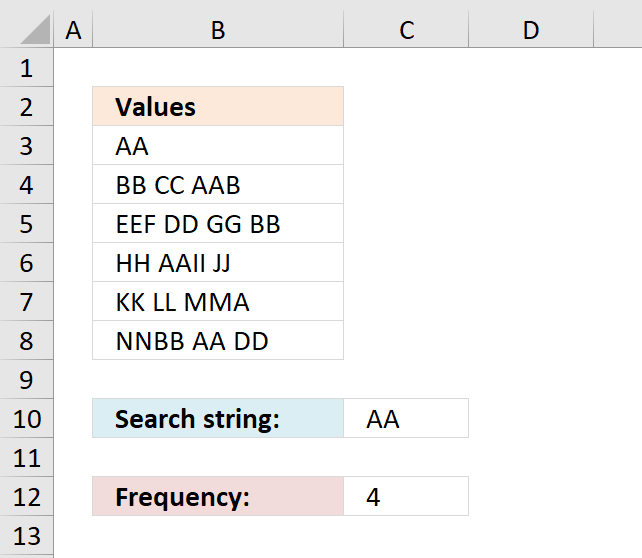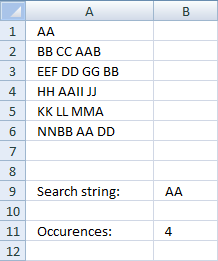Author: Oscar Cronquist Article last updated on October 15, 2018### Question:

How do I count the number of times a text string exists in a column? The text string may exist multiple times in a cell.### Array formula in cell B11:

=(SUM(LEN(tbl))-SUM(LEN(SUBSTITUTE(tbl, \$B\$9, ""))))/LEN(\$B\$9)

How to create an array formula

1. Copy array formula (Ctrl + c)
2. Select cell B11
3. Paste array formula (Ctrl + v) to formula bar4. Press and hold Ctrl + Shift
5. Press Enter
6. Release all keys

You can also use this formula to count how many times a specific character exists in a column in excel.

### Explain array formula in cell B11

=(SUM(LEN(tbl))-SUM(LEN(SUBSTITUTE(tbl, \$B\$9, ""))))/LEN(\$B\$9)

Step 1 - Replace existing text strings with new text string in named range tbl (A1:A6)

=(SUM(LEN(tbl))-SUM(LEN(SUBSTITUTE(tbl, \$B\$9, ""))))/LEN(\$B\$9)

Substitute(text, old_text, new_text, [instance_num]) replaces existing text with new text in a text string

SUBSTITUTE(tbl, \$B\$9, "")

becomes

SUBSTITUTE({"AA";"BB CC AAB";"EEF DD GG BB";"HH AAII JJ";"KK LL MMA";"NNBB AA DD"}, "AA", "")

returns this array:

{"";"BB CC B";"EEF DD GG BB";"HH II JJ";"KK LL MMA";"NNBB  DD"}

Step 2 - Return the number of characters in the named range tbl (A1:A6) without text string "AA"

=(SUM(LEN(tbl))-SUM(LEN(SUBSTITUTE(tbl, \$B\$9, ""))))/LEN(\$B\$9)

SUM(LEN(SUBSTITUTE(tbl, \$B\$9, "")))

becomes

SUM(LEN({"";"BB CC B";"EEF DD GG BB";"HH II JJ";"KK LL MMA";"NNBB  DD"}))

and returns 44

Step 3 - Return the number of characters in the named range tbl (A1:A6)

=(SUM(LEN(tbl))-SUM(LEN(SUBSTITUTE(tbl, \$B\$9, ""))))/LEN(\$B\$9)

SUM(LEN(tbl))

becomes

SUM(LEN({"AA";"BB CC AAB";"EEF DD GG BB";"HH AAII JJ";"KK LL MMA";"NNBB AA DD"}))

becomes

SUM({2;9;12;10;9;10})

returns 52.

Step 4 - Return the number of characters in cell B9

(SUM(LEN(tbl))-SUM(LEN(SUBSTITUTE(tbl, \$B\$9, ""))))/LEN(\$B\$9)

LEN(\$B\$9)

becomes

LEN("AA")

returns 2.

Step 5 - All together

=(SUM(LEN(tbl))-SUM(LEN(SUBSTITUTE(tbl, \$B\$9, ""))))/LEN(\$B\$9)

becomes

=(52-44)/2)

becomes

=8/2

returns 4.

Named ranges

tbl (A1:A6)

What is named ranges?

Get excel example file

count-text-string-in-a-column.xls

(Excel 97-2003 Workbook *.xls)